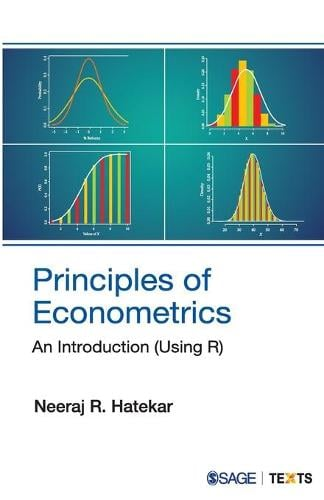•# Principles of Econometrics: An Introduction (Using R) (Paperback)

(author)
£30.00
Paperback 464 Pages / Published: 10/11/2010
• We can order this

Usually dispatched within 3 weeks

This textbook makes learning the basic principles of econometrics easy for undergraduate and postgraduate students of economics. It specifically caters to the syllabus of 'Introductory Econometrics' course taught in the third year of the Bachelor of Economics programme in many universities.

Principles of Econometrics takes the readers step-by-step from introduction to understanding, first introducing the basic statistical tools like concepts of probability, statistical distributions and hypothesis tests, and then going on to explain the two variable linear regression models along with certain additional tools such as the use of dummy variables and various data transformations. The most innovative feature of this textbook is that it familiarizes students with the role of R, which is a flexible and popular programming language. Using R, students will be able to implement a linear regression model and deal with the associated problems with substantial confidence.

Publisher: SAGE Publications India Pvt Ltd
ISBN: 9788132104698
Number of pages: 464
Weight: 440 g
Dimensions: 215 x 139 x 23 mm

MEDIA REVIEWS
Instead of presenting a traditional analysis of the capitalist system in integrating the real sector, the book combines Keynesian and Marxian approaches to the subject while unfolding the interrelation between the economy, State and society.

-- The Organiser